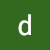# What is merge sort?

Merge sort is one of the most efficient sorting technique which was founded by John von Neumann in 1945. It is based on Divide and Conquer algorithm .It breaks down a problem into two or more sub-problems of the same type, till these become simple enough to get solved.

# Divide and conquer algorithm

In this approach, the problem is divided into sub-problems which are solved independently. After that the solution of sub-problems are finally merged up so that we can get the solution. It is divided in a 3 step process namely divide/break, conquer/solve and merge/combine.

# How merge sort works ?

1. If there is only one element present, return…CSE student